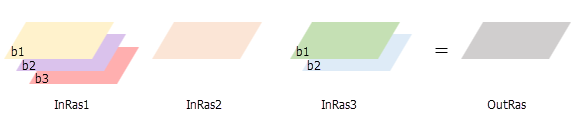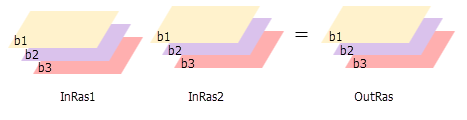# Cell Statistics function

## Overview

This function calculates statistics from multiple rasters, on a pixel-by-pixel basis. The available statistics are majority, maximum, mean, median, minimum, minority, range, standard deviation, sum, and variety.

## Notes

The order of the input rasters is irrelevant for this raster function.

If a single raster is used as the input for maximum, minimum, mean, median, majority, minority, or sum, the output pixel values will be the same as the input pixel values. If a single raster is used for range or standard deviation, the output cell values will all be 0. If a single raster is used for variety, the output pixel values will all be 1.

For each Operation, you can also choose to calculate the statistics by excluding all the NoData pixels.

To treat all bands from multiband inputs equally, uncheck the Process as multiband parameter. This means any multiband rasters will be converted into multiple single band rasters. The tool will then operate across all of the input bands, and return the result of the statistic being calculated on them as a single band output raster. To process a selection of bands from a multiband raster, first create a new raster dataset composed of those particular bands with the Composite Bands raster function, and then use it as input to the function.To calculate multiband output statistics from multiband input rasters, check the Process as multiband parameter. In this case, each multiband input will be used to calculate band-wise statistics. This means the statistic will be calculated for the first band across all the inputs, then the second band across all the inputs, and so on. The results from each band will be used to create a multiband output. In this case, all inputs must have equal number of bands. If some of your intended inputs have fewer bands than others, you will need to create new NoData bands to get equal number of bands for all inputs. You can do this by using the Composite Band raster function to create new multiband datasets, combining the existing bands with NoData for the missing bands. You can then use the resulting multiband rasters as inputs to the function.If all inputs are multidimensional rasters with same number of variables, the function will perform the operation for all slices with same dimension value, and the output will be a multidimensional raster. The variables in the inputs must have the same dimensions or common dimensions.

If one or more of the inputs is a multidimensional raster and the other input is a constant, the function will perform the operation for all slices for all variables against the constant value, and the output will be a multidimensional raster.

## Parameters

Parameter nameDescription

Rasters

(Required)

The list of rasters from which to calculate statistics, on a pixel-by-pixel basis.

Operation

The type of statistical calculation that you want to perform.

The available statistics are Majority, Maximum, Mean, Median, Minimum, Minority, Range, Standard Deviation, Sum, and Variety.

The default is Majority.

Cellsize Type

Choose which cell size to use in the output raster. If all the input cell sizes are the same, all the options will yield the same results.

• First Of—Use the first cell size of the input rasters.
• Min Of—Use the smallest cell size of all the input rasters.
• Max Of—Use the largest cell size of all the input rasters. This is the default.
• Mean Of—Use the mean cell size of all the input rasters.
• Last Of—Use the last cell size of the input rasters.

Extent Type

Choose which extent should be used in the output raster:

• First Of—Use the extent of the first input raster to determine the processing extent.

• Intersection Of—Use the extent of the overlapping pixels to determine the processing extent. This is the default.
• Union Of—Use the extent of all the rasters to determine the processing extent.
• Last Of—Use the extent of the last input raster to determine the processing extent.

Process as multiband

Determines how the input rasters bands are processed.

• Unchecked—All multiband inputs will be processed as single bands. This is the default.
• Checked—All multiband inputs will be processed as multiband.

Here is a list of all of the operations and a description for each of them. For more information about each of the operations, see How Cell Statistics works.

Parameter nameDescription

Majority

Determines the value that occurs most often on a pixel-by-pixel basis.

Maximum

Determines the largest value on a pixel-by-pixel basis.

Mean

Calculates the average on a pixel-by-pixel basis.

Median

Calculates the middle value of the pixels on a pixel-by-pixel basis.

Minimum

Determines the smallest value on a pixel-by-pixel basis.

Minority

Determines the value that occurs least often on a pixel-by-pixel basis.

Range

Calculates the difference between the largest and the smallest value on a pixel-by-pixel basis.

Standard Deviation

Calculates the standard deviation of the pixels on a pixel-by-pixel basis.

Sum

Calculates the total value on a pixel-by-pixel basis.

Variety

Calculates the number of unique values on a pixel-by-pixel basis.

Majority (Ignore NoData)

Determines the value that occurs most often on a pixel-by-pixel basis.

Only cells that have data values will be used in determining the statistic value. If a NoData value exists at a certain location, the NoData value will be ignored.

Maximum (Ignore NoData)

Determines the largest value on a pixel-by-pixel basis.

Only cells that have data values will be used in determining the statistic value. If a NoData value exists at a certain location, the NoData value will be ignored.

Mean (Ignore NoData)

Calculates the average on a pixel-by-pixel basis.

Only cells that have data values will be used in determining the statistic value. If a NoData value exists at a certain location, the NoData value will be ignored.

Median (Ignore NoData)

Calculates the middle value of the pixels on a pixel-by-pixel basis.

Only cells that have data values will be used in determining the statistic value. If a NoData value exists at a certain location, the NoData value will be ignored.

Minimum (Ignore NoData)

Determines the smallest value on a pixel-by-pixel basis.

Only cells that have data values will be used in determining the statistic value. If a NoData value exists at a certain location, the NoData value will be ignored.

Minority (Ignore NoData)

Determines the value that occurs least often on a pixel-by-pixel basis.

Only cells that have data values will be used in determining the statistic value. If a NoData value exists at a certain location, the NoData value will be ignored.

Range (Ignore NoData)

Calculates the difference between the largest and the smallest value on a pixel-by-pixel basis.

Only cells that have data values will be used in determining the statistic value. If a NoData value exists at a certain location, the NoData value will be ignored.

Standard Deviation (Ignore NoData)

Calculates the standard deviation of the pixels on a pixel-by-pixel basis.

Only cells that have data values will be used in determining the statistic value. If a NoData value exists at a certain location, the NoData value will be ignored.

Sum (Ignore NoData)

Calculates the total value on a pixel-by-pixel basis.

Only cells that have data values will be used in determining the statistic value. If a NoData value exists at a certain location, the NoData value will be ignored.

Variety (Ignore NoData)

Calculates the number of unique values on a pixel-by-pixel basis.

Only cells that have data values will be used in determining the statistic value. If a NoData value exists at a certain location, the NoData value will be ignored.